# NCERT Solutions for Class 6 Maths Chapter 4 Basic Geometrical Ideas

## NCERT Solutions for Class 6 Maths Chapter 4 Basic Geometrical Ideas

Today in our article you will be told that when your ncert solutions for class 6 maths Chapter 4 Basic Geometrical Ideas will be released and you will be provided complete information about it. In our article, you will also be given complete information about the model paper. Also, you will be provided complete information about the model paper download process in our article. So please read it carefully till the end and stay connected with our website till the end.

ncert solutions for class 6 maths Chapter 4

## Exercise 4.1

1. Use the figure to name:

(a) Five points

(b) A line

(c) Four rays

(d) Five line segments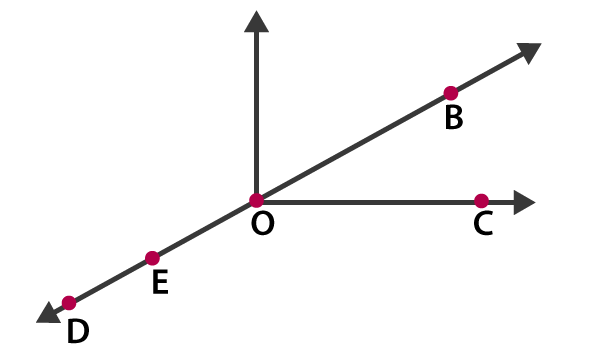Solutions:

(a) The five points are D, E, O, B and C

(b) A line is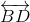(c) Four rays are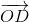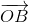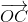and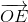.

(d) Five line segments are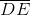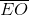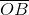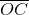and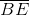2. Name the line given in all possible (twelve) ways, choosing only two letters at a time from the four given.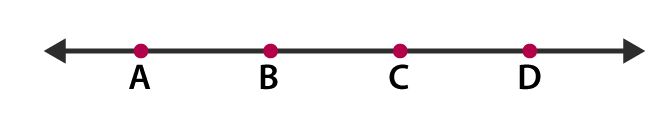Solutions:

The lines are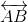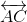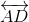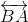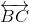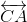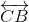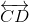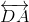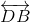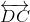3. Use the figure to name:

(a) Line containing point E.

(b) Line passing through A.

(c) Line on which O lies

(d) Two pairs of intersecting lines.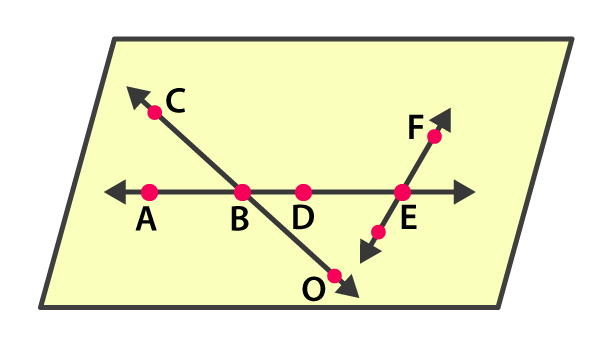Solutions:

(a) Line containing point E is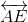(b) Line passing through A is(c) Line on which O lies is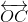(d) Two pairs of intersecting lines are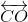and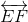4. How many lines can pass through (a) one given point? (b) two given points?

Solutions:

(a) Countless lines can pass through a given point.

(b) Only one line can pass through two given points.

5. Draw a rough figure and label suitably in each of the following cases:

(a) Point P lies on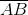.

(b)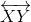and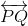intersect at M.

(c) Line l contains E and F but not D.

(d)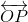and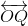meet at O.

Solutions:

(a)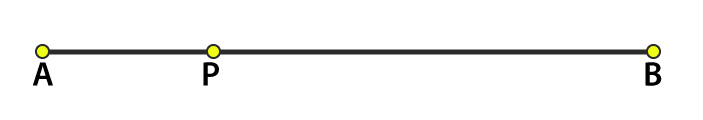(b)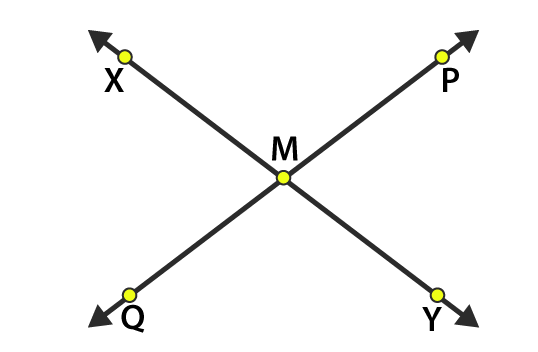(c)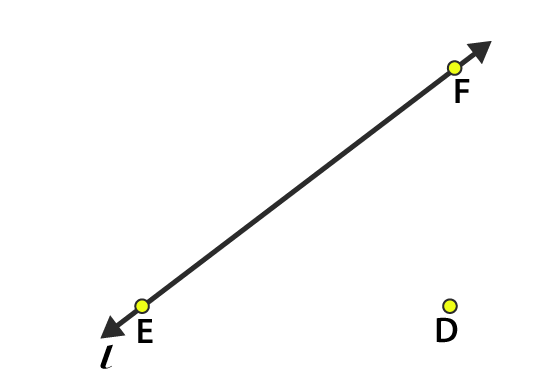(d)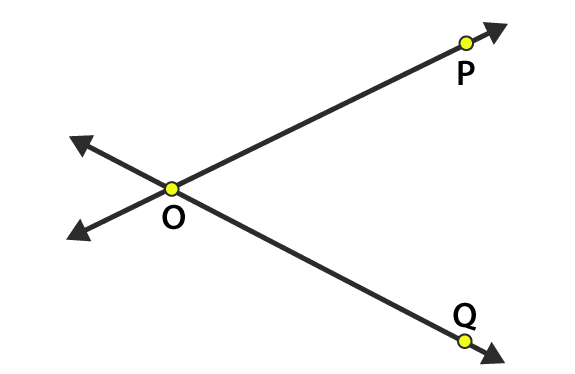6. Consider the following figure of line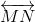. Say whether the following statements are true or false in the context of the given figure.

(a) Q, M, O, N, P are points on the line.

(b) M, O, N are points on a line segment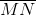.

(c) M and N are end points of line segment.

(d) O and N are end points of line segment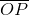.

(e) M is one of the end points of line segment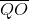.

(f) M is point on ray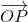.

(g) Rayis different from ray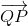.

(h) Rayis the same as ray.

(i) Ray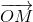is not opposite to ray.

(j) O is not an initial point of(k) N is the initial point of and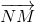.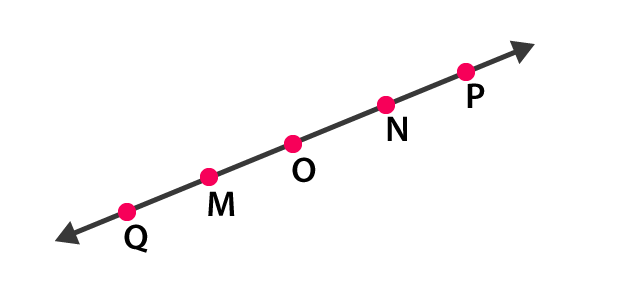Solutions:

(a) True

(b) True

(c) True

(d) False

(e) False

(f) False

(g) True

(h) False

(i) False

(j) False

(k) True

## Exercise 4.2

1. Classify the following curves as (i) Open or (ii) Closed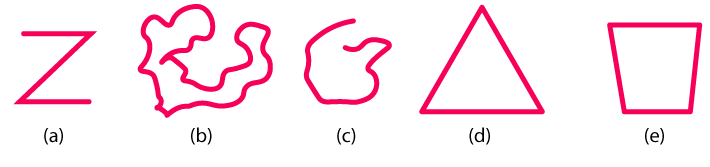Solutions:

(a) The given curve is an open curve

(b) The given curve is closed curve

(c) The given curve is an open curve

(d) The given curve is closed curve

(e) The given curve is closed curve

2. Draw rough diagrams to illustrate the following:

(a) Open curve

(b) Closed curve

Solutions

(a) The below figure is the open curve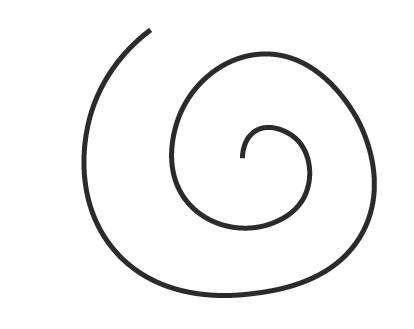(b) The below figure is the closed curve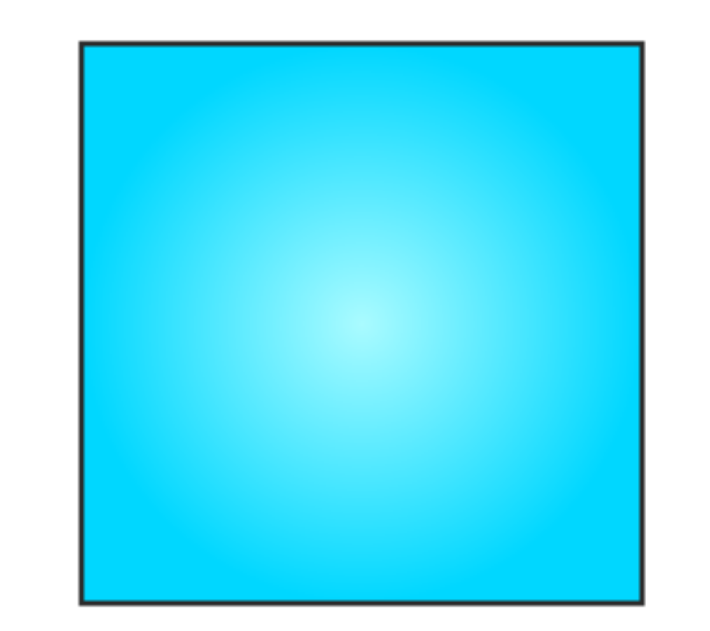3. Draw any polygon and shade its interior.

Solutions:

The below figure is the polygon with interior shade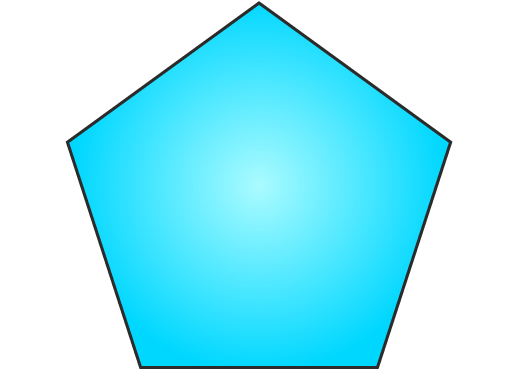4. Consider the given figure and answer the questions:

(a) Is it a curve?

(b) Is it closed?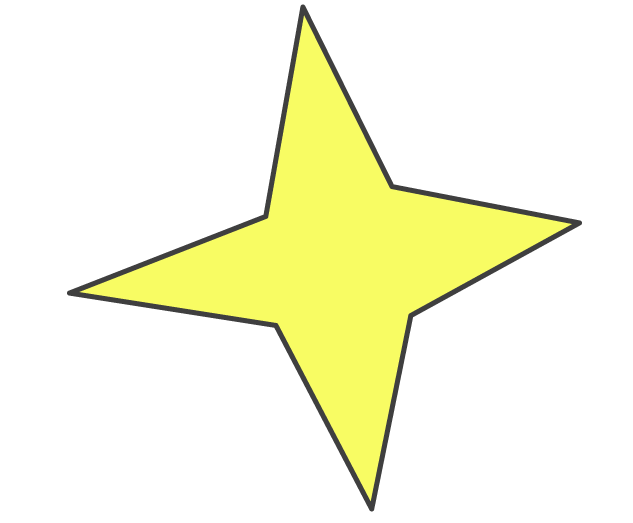Solutions:

(a) Yes, it is a curve

(b) Yes, it is a closed curve

5. Illustrate, if possible, each one of the following with a rough diagram:

(a) A closed curve that is not a polygon.

(b) An open curve made up entirely of line segments.

(c) A polygon with two sides.

Solutions:

(a) The below figure is a closed figure but not a polygon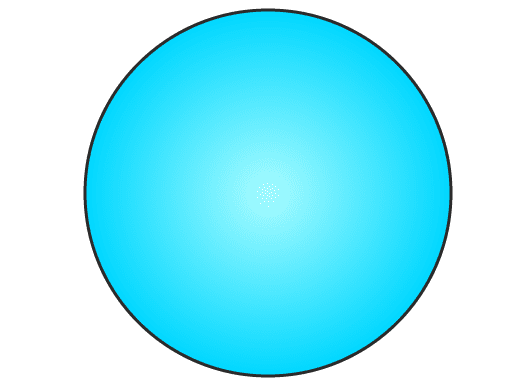(b) The below figure is an open curve made up entirely of line segments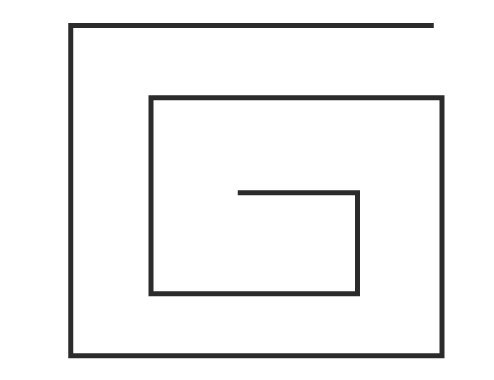(c) No it’s not possible, as the polygon having the least number of sides is a triangle that has three sides.

## Exercise 4.3

1. Name the angles in the given figure.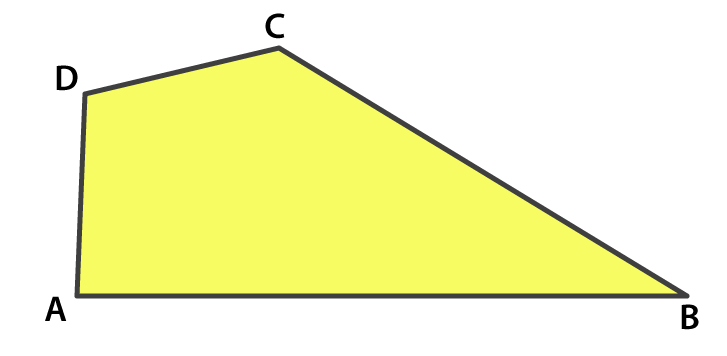Solutions:

The angles are ∠DAB, ∠ABC, ∠BCD, and ∠CDA

2. In the given diagram, name the points(s)

(a) In the interior of ∠DOE

(b) In the exterior of ∠EOF

(c) On ∠EOF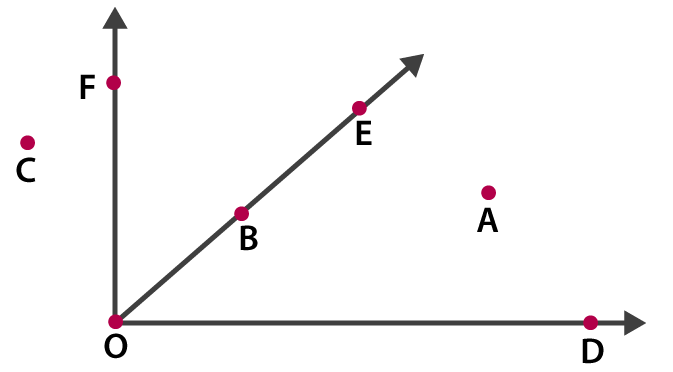Solutions:

(a) The point in the interior of ∠DOE is A

(b) The point in the exterior of ∠EOF is C, A and D

(c) The points on ∠EOF are E, B, O, and F

3. Draw rough diagrams of two angles such that they have

(a) One point in common

(b) Two points in common

(c) Three points in common

(d) Four points in common

(e) One ray in common

Solutions:

(a) O is the common point between ∠COD and ∠AOB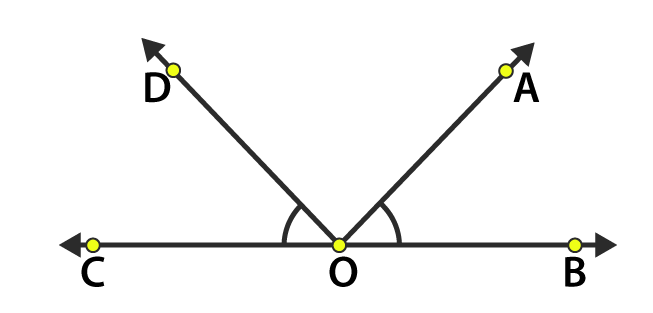(b) O and B are common points between ∠AOB and ∠BOC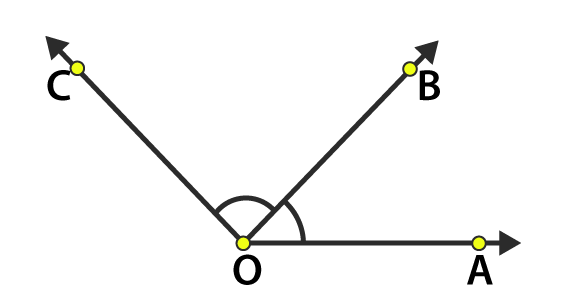(c) O, E and B are common points between ∠AOB and ∠BOC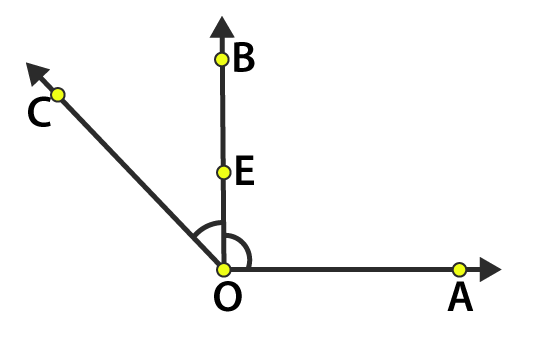(d) O, E, D, and A are common points between ∠BOA and ∠COA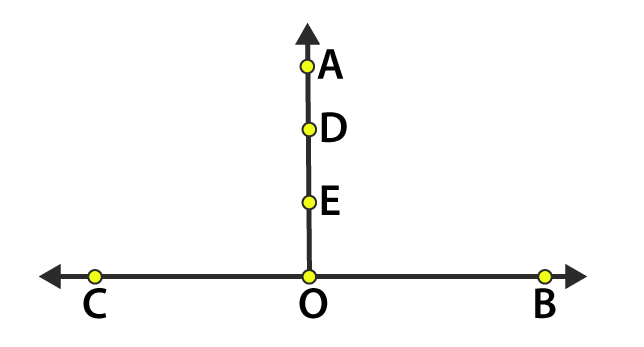(e) OC is common ray between ∠BOC and ∠AOC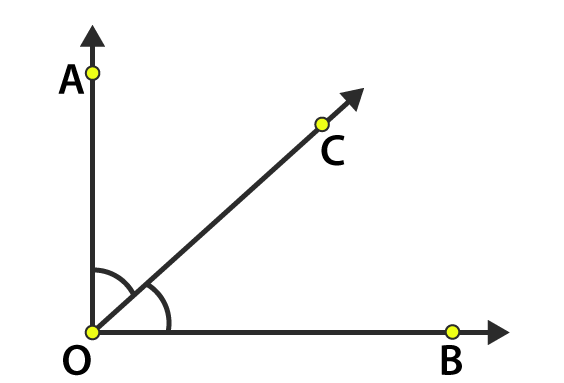## Exercise 4.4

1. Draw a rough sketch of a triangle ABC. Mark a point P in its interior and a point Q in its exterior. Is point A in its exterior or in its interior?

Solutions:

Point A lies on the given triangle ABC. It lies neither in interior nor exterior.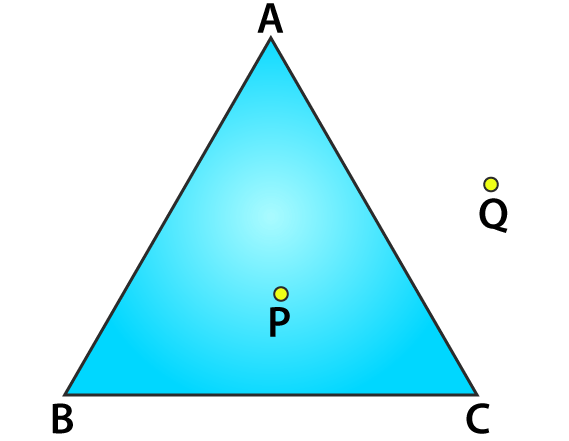2. (a) Identify three triangles in the figure.

(b) Write the names of seven angles.

(c) Write the names of six line segments

(d) Which two triangles have ∠B as common?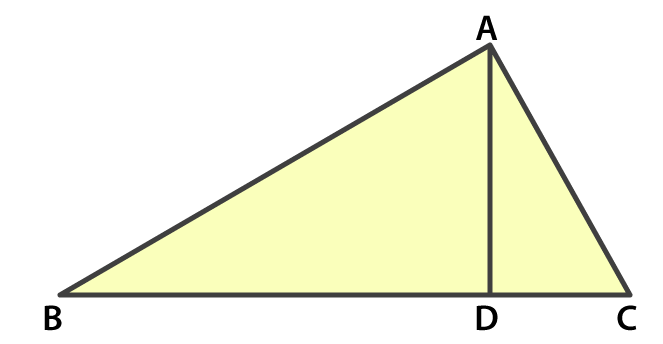Solutions:

(a) The three triangles are ∠ABD, ∠ACB, ∠ADC

(c) The line segments are,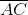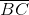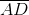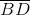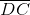(d) ∠ABD and ∠ABC are triangles that have ∠B as common.

## Exercise 4.5

1. Draw a rough sketch of a quadrilateral PQRS. Draw its diagonals. Name them. Is the meeting point of the diagonals in the interior or exterior of the quadrilateral?

Solutions:

PR and QS are the diagonalsThey meet at point O which is in the interior of the quadrilateral.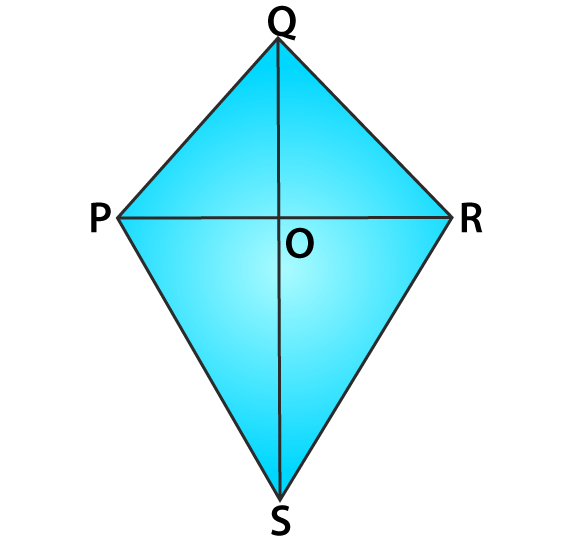2. Draw a rough sketch of a quadrilateral KLMN. State,

(a) two pairs of opposite sides,

(b) two pairs of opposite angles,

(c) two pairs of adjacent sides,

(d) two pairs of adjacent angles.

Solutions: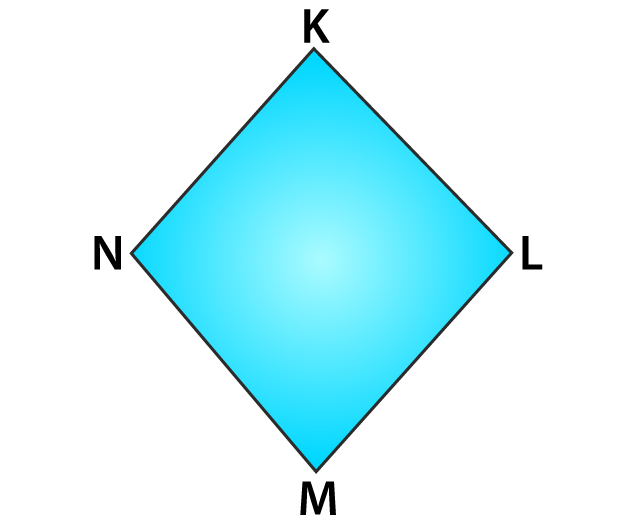(a) Two pairs of opposite sides are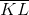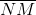and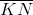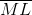(b) Two pairs of opposite angles are ∠KLM, ∠KNM, and ∠LKN, ∠LMN

(c) Two pairs of adjacent sides areand,or,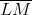and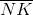(d) Two pairs of adjacent angles are ∠K, ∠L and ∠M, ∠N or ∠K, ∠L, and ∠L, ∠M

Exercise 4.6 page no: 84

1. From the figure, identify:

(a) the centre of the circle

(c) a diameter

(d) a chord

(e) two points in the interior

(f) a point in the exterior

(g) a sector

(h) a segment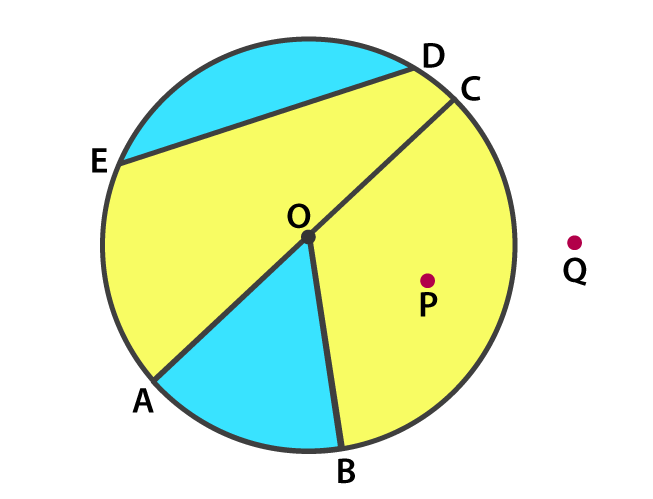Solutions:

(a) The centre of the circle is O

(b) Three radii are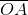(c) A diameter is(d) A chord is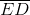(e) Two points in the interior are O and P

(f) A point in the exterior is Q

(g) A sector is AOB i.e shaded region

(h) A segment is ED i.e shaded region

2. (a) Is every diameter of a circle also a chord?

(b) Is every chord of a circle also a diameter?

Solutions:

(a) Yes every diameter of a circle is also a chord. Diameter is also called as a longest chord.

(b) No, every chord is not a diameter.

3. Draw any circle and mark

(a) it’s centre

(c) a diameter

(d) a sector

(e) a segment

(f) a point in its interior

(g) a point in its exterior

(h) an arc

Solutions: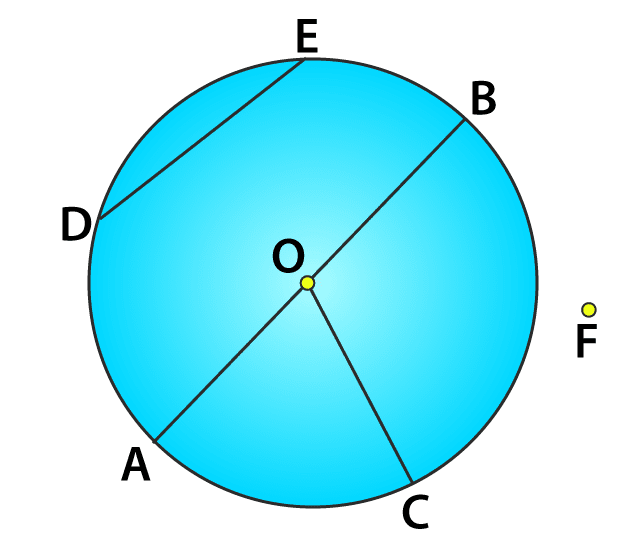(a) The centre of the circle is O.

(c) A diameter is(d) A sector is AOC

(e) A segment is DE

(f) A point in its interior is O

(g) A point in its exterior is F

(h) An arc is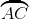4. Say true or false:

(a) Two diameters of a circle will necessarily intersect.

(b) The centre of a circle is always in its interior.

Solutions:

(a) True, two diameters will always intersect each other at the centre of the circle.

(b) True, the centre of the circle will always be in its interior.

• Chapter 1 Knowing our Numbers
• Chapter 2 Whole Numbers
• Chapter 3 Playing With Numbers
• Chapter 4 Basic Geometrical Ideas
• Chapter 5 Understanding Elementary Shapes
• Chapter 6 Integers
• Chapter 7 Fractions
• Chapter 8 Decimals
• Chapter 9 Data Handling
• Chapter 10 Measurement And Mensuration
• Chapter 11 Algebra
• Chapter 12 Ratio Proportion
• Chapter 13 Introduction To Symmetry
• Chapter 14 Practical Geometry Application

I hope you like the information and notes given by us. This will help you a lot in your upcoming exams. Here we are giving ncert solutions to all of you students, through which all of you can score very well in your exams. In this post, we are giving you ncert solutions for class 6 maths. hope you benefit from it. You can download the pdf file of this ncert solutions.

 Whatsapp Group Join
 Telegram channel Join